# What Is Perimeter Used For? (10 Real Life Uses Of Perimeter)

Perimeter is used often in mathematics for word problems in geometry and optimization problems in calculus. Perimeter is vital for finding the surface area of some objects, but it also has numerous applications in real life.

So, what is perimeter used for?  Perimeter is used for various applications in business, housing, construction, gardening, and crafts. For example, a gardener uses perimeter to find out how much fence to use to close in a rectangular garden. A painter uses perimeter to help figure out how much paint to buy for a job.

Of course, perimeter has many more applications in real life: from area optimization to buildings, perimeter plays a role.

In this article, we’ll talk about 10 important real life uses of perimeter.  We’ll also give some examples so you can see the numbers at work.

Let’s get started.

## What Is Perimeter Used For? (10 Uses Of Perimeter)

Perimeter has many applications in business, housing, construction, gardening, and crafts, and other disciplines.  Here are 10 ways that perimeter is used in real life:

• Area Optimization (limited materials)
• Border Lengths For States & Countries
• Building A Shed
• Gardening (length of fence needed to enclose an area)
• Molding Above Doors & Windows
• Picture Frames
• Surveying (Real Estate Property Lines)
• Paint or Wallpaper For A Room
• Running On A Track (distance around)
• Upholstery (Furniture Repair)

Let’s take a closer look at each of these in turn, starting with business.

### Area Optimization

Perimeter helps us to minimize the use of materials when we want a shape with a certain area.  You can solve some of these problems by graphing, but others may require calculus.

#### Example: Using Perimeter To Optimize Area

Let’s say that you want a rectangular cardboard box with an area of 36 square inches.  We want to find the dimensions (length and width) that minimize the perimeter.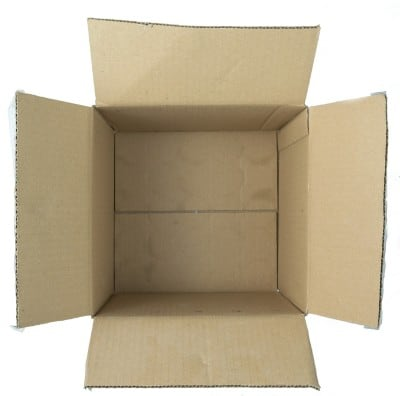A cardboard box with a rectangular base will have the maximum area for a given perimeter when the length and width are equal (that is, a square base).

This will minimize the amount of cardboard used to make the box, thus reducing waste.

First, we will name variables: call the dimensions x (length) and y (width).

Next, come up with an equation for total perimeter of the box.  Since each side is counted twice, we get a perimeter of:

• P = 2x + 2y

Then, we come up with an equation to represent a constraint on the dimensions.  Specifically, the area must be 36 square inches.  The area of a rectangle with sides x and y is xy, so we get:

• xy = 36

Now, we must minimize the perimeter by using the constraint.  To make the problem easier, we will rewrite P = 2x + 2y so that it is a function of one variable instead of two.

Solving xy = 36 for y, we get:

• y = 36 / x

Substituting y into P = 2x + 2y, we get:

• P = 2x + 2y
• P = 2x + 2(36 / x)
• P(x) = 2x + (72 / x)

We want to find the value of x that minimizes the function P(x) above.  We can graph the function (shown below) or we can use calculus to find the minimums by using derivatives.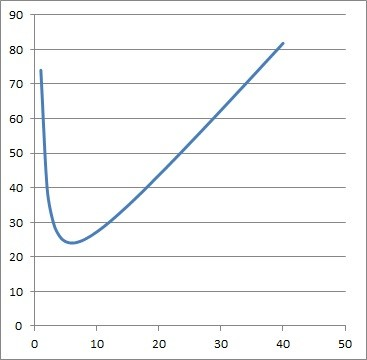The graph of the function p(x) = 2x + (72 / x) has a minimum at x = 6.

The first derivative is

• P’(x) = 2 – (72 / x2)

The first derivative is zero when:

• P’(x) = 0
• 2 – (72 / x2) = 0
• 2 = 72 / x2
• 2x2 = 72
• X2 = 36
• x = +/- 6

Since x is a length, we want the positive value, so x = 6.

We can verify that x = 6 is a minimum for the perimeter function by taking the second derivative:

• P’’(x) = 144 / x3

Which is positive when x = 6, meaning we have a minimum at that point.

Since x = 6, we must also have y = 6, since y = 36 / x.

So, the box should have a length of 6 inches and a width of 6 inches to have an area of 36 square inches.  We can find the volume of the box if we multiply the area by the height.

### Border Lengths

The perimeter of a shape tells us the distance you would need to walk to go all the way around the shape.  So, the perimeter of a state or country tells us the length of a border.

You can also find the part of a state or country’s border that touches an ocean.  This can give you an idea of how much of the border is susceptible to tidal waves, tsunamis, and storms from the ocean.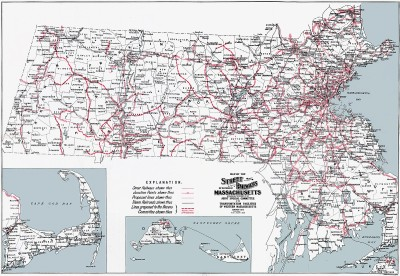You can use a state map to find out how much of the border touches the ocean.

### Building A Shed

If you want to build a wooden shed, you need to know how much lumber to buy.  The perimeter of the shed is one thing you will need to calculate before you begin working.

The perimeter of the shed tells you the board-length that you will need for the walls.  For example, if the shed has a height of 8 feet, a length of 10 feet, and width of 12 feet, then the perimeter is:

• Perimeter = 2*length + 2*width
• Perimeter = 2*10 + 2*12
• Perimeter = 44 feet

So you would need 44 feet of board that is 8 feet tall to build the perimeter of the shed.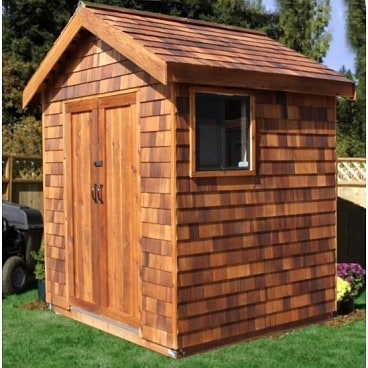Finding the perimeter of a shed helps you to figure out how much wood you will need to build it.

### Gardening

When building a fence for a garden to keep animal pests out, you will need to know how much fencing to buy.  The perimeter will tell you this.

For example, if the garden is 20 feet long by 30 feet wide, then the perimeter is:

• Perimeter = 2*length + 2*width
• Perimeter = 2*20 + 2*30
• Perimeter = 100 feet

So you would need 100 feet of fence to close in the entire garden.  Of course, you will also need one or two gates so you can get in and out easily.

### Molding

In construction, molding is used around doors and windows as decoration.  To find out how much molding you will need for a window, you will need to calculate the perimeter of a window.

For a door, you will need to calculate the perimeter without the bottom part (since molding does not go at the bottom of a door).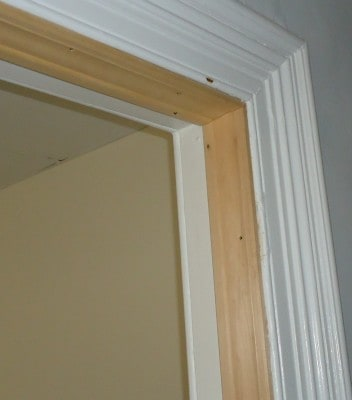The perimeter of a doorway (without the bottom side) tells you how much molding you will need to surround it.

### Picture Frames

When making picture frames, you need to know how much material you need to go all the way around the picture.  As before, the perimeter is twice the length plus twice the width.

For example, if the picture is 8 inches long by 10 inches wide, then the perimeter is:

• Perimeter = 2*length + 2*width
• Perimeter = 2*8 + 2*10
• Perimeter = 36 inches

So, a piece of material (wood, plastic, metal) that is 36 inches long (3 feet) will give you enough material to make one frame.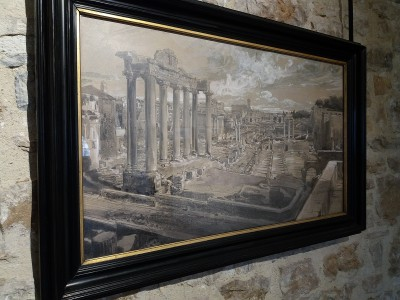Knowing the perimeter of a picture frame helps us to figure out how much material we need to build the frame.

### Surveying

In property surveying, the goal is to figure out where one property ends and another begins.  Part of this process involves finding the dimensions of a piece of land.

A piece of land could have 3, 4, or more sides.  It can also have sharp corners (or at least corners that are not 90 degrees).

If you were to add up all of the side lengths, you could find the perimeter of that piece of land.

For example, consider the piece of land with dimensions pictured below.

The perimeter would be:

• 60 + 72 + 90 + 108 + 120 = 450 feet

So you would need to walk 450 feet to go around the entire perimeter.  This means you would also need 450 linear feet of fencing to go around the entire perimeter.If you want to build a fence all the way around a property, you will need to know the perimeter.

### Paint & Wallpaper

When painting or wallpapering the walls in a room, you can use the perimeter (along with the height) to help you figure out how much paint or wallpaper you need.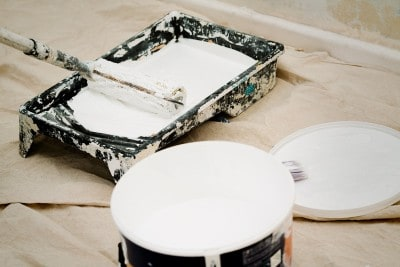Knowing the perimeter of a room helps you to calculate how much paint or wallpaper you need for full coverage.

#### Example: Using Perimeter To Find Area For Painting Or Wallpaper

Let’s say that you have a room with a length of 15 feet, a width of 14 feet, and a height of 10 feet.  You are going to paint (or wallpaper) the walls, but not the ceiling.

So, you will need to know how much area you need to cover.  First, we find the perimeter of the room, which is:

• Perimeter = 2*length + 2*width
• Perimeter = 2*15 + 2*14
• Perimeter = 58 feet

Now, we multiply by the height to get the area of the four walls.  This gives us:

• Surface Area Of Walls = Perimeter*Height
• Surface Area Of Walls = 58*10
• Surface Area Of Walls = 580 square feet

So, you have 580 square feet of wall to cover with paint or wallpaper (this ignores space for the door or window, so you might have a little extra).

Since a can of paint covers about 400 square feet, you would need 580 / 400, or about 1.5 cans of paint.  You would buy 2 cans and have some left over for touch-up or repair work later on.

### Running On A Track

When running around a track, perimeter tells you how much distance you cover every time you go around.  Assuming a circular track, we would use circumference (which is the perimeter of a circle).The perimeter of a track tells you how far you need to walk or run to go around the track once.

#### Example: Perimeter Of A Circular Track

You want to know the distance around a circular track at a college.  The track is 200 feet from the center to an edge (radius = 200 feet).

Then the perimeter (circumference) of the circular track is:

• Circumference = 2*π*R [R = radius of circle]
• Circumference = 2*π*200
• Circumference = 400*π
• Circumference = 1257 feet

So, one time around the track is 1257 feet – just under a quarter of a mile (which is 1320 feet).

### Upholstery

In upholstery (furniture repair), we might need to figure out the perimeter of a shape if we want to add a certain type of border all around the shape.

The perimeter would tell us how much of the border material we need to go all the way around the shape.

## Conclusion

Now you know some of the uses of perimeter in real life, along with how to do some of the calculations for those applications.

You might also want to check out my article on how circles are used.

You can learn about various uses of algebra in real life here.

You can learn how to find the perimeter of a square here.